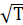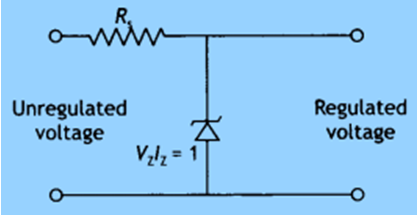Daily Practice Problems
Class 12 Physics
Semiconductor Electronics: Material Devices and Simple Circuits­Question 1:

Which among the following is the most commonly used semiconductor?

(a) Silicon

(b) Carbon

(c) Germanium

(d) Sulphur

Question 2:

Which of the following is compound semiconductor?

(a)CdS

(b)CdAs

(c)InP

(d)All of these

Question 3:

Which of the following is created when trivalent impurities are added to a semiconductor?

(a) Free electrons

(b) Holes

(c) Bound electrons

(d) Valence electrons

Question 4:

The relation between the number of free electrons in semiconductor and its temperature is given as

(a)T

(b) nαT2

(c) nαT3/2

(d)Question 5:

Which of the following diode is used in voltage regulator?

(a) Photo diode

(b) Zener diode

(c) LED

(d) p-n junction diode

Question 6:

Which material has a negative temperature coefficient?

(a) Carbon

(b) Aluminium

(c) Brass

(d) Copper

Question 7:

A semiconductor placed at 0k, it will behave as

(a) conductor

(b) insulator

(c) metal

(d) semiconductor

Question 8:

The forbidden energy gap for germanium is

(a) 0.12 eV

(b) 0.72 eV

(c) 1.11 eV

(d) 1.52 eV

Question 9:

In a middle of depletion layer of a reverse biased p-n junction, the

(a) electric field is zero

(b) potential is maximum

(c) electric field is maximum

(d) potential is 0

Question 10:

The output of OR gate is 1 when

(a) if both the inputs are 0

(b) if either or both inputs are 1

(c) only if both inputs are 1

(d) if either input 0

Question 11:

Why should a photodiode be operated at a reverse bias?

Question 12:

Draw the voltage-current characteristic for a Zener diode.

Question 13:

Suppose a pure Si crystal has 5 × 1028 atoms m–3. It is doped by 1 ppm concentration of pentavalent As. Calculate the number of electrons and holes. Given that ni =1.5 × 1016 m–3.

Question 14:

Explain briefly, which property of the characteristic enables us to use Zener diode as voltage regulator.

Question 15:

Draw the energy band diagram of n-type and p-type semiconductors.

Question 16:

A Zener of power rating 1 W is to be used as a voltage regulator. If Zener has a breakdown of 5 V and it has to regulate voltage which fluctuated between 3 V and 7 V, what should be the value of R, for safe operation (figure)?Question 17:

Define the terms ‘potential barrier’ and ‘depletion region’ for a p – n junction diode. State how the thickness of the depletion region will change when the p-n junction diode is (i) forward biased and (ii) reverse biased.

Question 18:

With the help of a diagram, show the biasing of a light-emitting diode (LED). Give its two advantages over conventional incandescent lamps.

Question 19:

Draw the circuit diagram of a full-wave rectifier and explain its working. Also, give the input and output waveforms.

Question 20:

Distinguish between conductors, insulators, and semiconductors on the basis of the band theory of solids.

**********